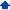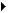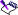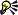### Creating frequency distribution tables

A frequency distribution table indicates the values that a variable can take on and the frequency with which each value occurs. For example, you can create a frequency distribution table to find the number of times that numbers between two and five appear in a given block of cells.

You can supplement the statistical information in your notebook with appropriate spreadsheet functions. For example, you can include these formulas at the end of the weekly sales figures contained in a selection named SALES.

 @SUM(SALES) Calculates total sales @COUNT(SALES) Calculates the number of sales in the column @AVG(SALES) Calculates the average sales amount

To create a frequency distribution table, you need one or more areas of values to count and bin cells listing the value intervals, or bins, for each count you need. Bin cells contain the range of intervals you want analyzed. The bin must be a single column with a column of blank cells to its right, where the results will be written. Value cells must also be placed in a single column.

#### To create a frequency distribution table1 Type values in a bin of ranges on the spreadsheet.
 2 Click ToolsNumeric toolsFrequency.
 3 Click the Range pickerin the Value cells box, and select the value cells.
 4 Click the Range pickerin the Bin cells box, and select the bin cells.
 The bin cell ranges must be in ascending order.• Each value in the bin cells represents all values from it down to the previous value. The first value represents any value less than or equal to itself.
 • The result area is one cell longer than the bin cells, and is displayed to the right of the bin cells. The last cell contains the number of values found that were greater than the final number in the bin.• To create an XY chart of a frequency distribution, specify the bin cells as the x-axis series and the results as the first series of values.